Request a Free Counselling Session from our Expert Mentor# NCERT Solution for Class 10th Maths Chapter 9 Some Applications Of Trigonometry

In this chapter, you will explore various real-world applications of trigonometry, such as finding the heights and distances of objects, determining angles of elevation and depression, and more. By using the concepts of trigonometry, you can solve practical problems related to heights and distances of objects, which can be beneficial in fields such as construction, architecture, and engineering.
This chapter has a total of 6 exercises, which cover various types of problems related to the applications of trigonometry. To help you understand the concepts and solve the problems effectively, we have provided step-by-step solutions for all the exercises. These solutions are designed in a way that is easy to understand and follow, and will help you gain confidence in applying trigonometry to real-world problems.
Overall, this chapter will help you develop a deeper understanding of trigonometry and its applications, and prepare you for more advanced topics in mathematics.

## Answers of Math NCERT solutions for Class 10th Maths Chapter 9 Some Applications Of Trigonometry

Unit 9

Some Applications of Trigonometry

Exercise 9.1

Q1 :

A circus artist is climbing a 20 m long rope, which is tightly stretched and tied from the top of a vertical pole to the ground. Find the height of the pole, if the angle made by the rope with the ground level is 30 °.It can be observed from the figure that AB is the pole. In ΔABC,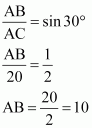Therefore, the height of the pole is 10 m.

Q2 :

A tree breaks due to a storm and the broken part bends so that the top of the tree touches the ground making an angle 30 ° with it. The distance between the foot of the tree to the point where the top touches the ground is 8 m. Find the height of the tree.

Let AC was the original tree. Due to storm, it was broken into two parts. The broken part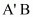is making 30° with the ground.

In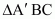,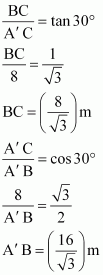Height of tree =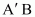+ BC

Hence, the height of the tree is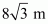.

Q3 :

A contractor plans to install two slides for the children to play in a park. For the children below the age of 5 years, she prefers to have a slide whose top is at a height of 1.5 m, and is inclined at an angle of 30 ° to the ground, where as for the elder children she wants to have a steep side at a height of 3 m, and inclined at an angle of 60 ° to the ground. What should be the length of the slide in each case?

It can be observed that AC and PR are the slides for younger and elder children respectively.

In ΔABC,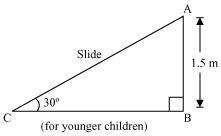In ΔPQR,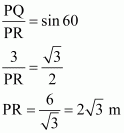Therefore, the lengths of these slides are 3 m and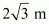.

Q4 :

The angle of elevation of the top of a tower from a point on the ground, which is 30 m away from the foot of the tower is 30°. Find the height of the tower.

Answer :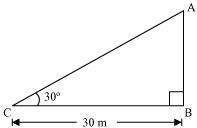Let AB be the tower and the angle of elevation from point C (on ground) is 30°.

In ΔABC,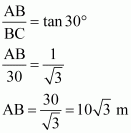Therefore, the height of the tower is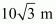.

*Q5 :

A kite is flying at a height of 60 m above the ground. The string attached to the kite is temporarily tied to a point on the ground. The inclination of the string with the ground is 60°. Find the length of the string, assuming that there is no slack in the string.

Answer :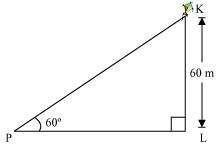Let K be the kite and the string is tied to point P on the ground. In ΔKLP,

Hence, the length of the string is    .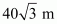Q6 :

A 1.5 m tall boy is standing at some distance from a 30 m tall building. The angle of elevation from his eyes to the top of the building increases from 30° to 60° as he walks towards the building. Find the distance he walked towards the building.

Answer :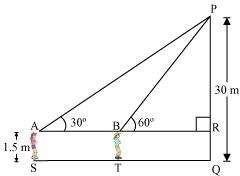Let the boy was standing at point S initially. He walked towards the building and reached at point T. It can be observed that

PR = PQ – RQ

= (30 – 1.5) m = 28.5 m =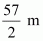In ΔPAR,

In ΔPRB,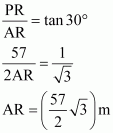ST = AB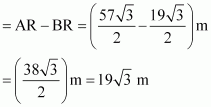Hence, he walked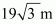towards the building.

*Q7 :

From a point on the ground, the angles of elevation of the bottom and the top of a transmission tower fixed at the top of a 20 m high building are 45° and 60° respectively. Find the height of the tower.

Answer :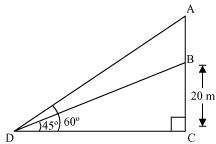Let BC be the building, AB be the transmission tower, and D be the point on the ground from where the elevation angles are to be measured.

In ΔBCD,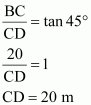In ΔACD,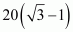Therefore, the height of the transmission tower is    m.

Q8 :

A statue, 1.6 m tall, stands on a top of pedestal, from a point on the ground, the angle of elevation of the top of statue is 60° and from the same point the angle of elevation of the top of the pedestal is 45 °. Find the height of the pedestal.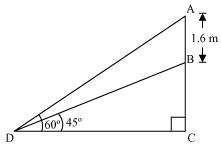Let AB be the statue, BC be the pedestal, and D be the point on the ground from where the elevation angles are to be measured.

In ΔBCD,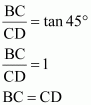In ΔACD,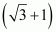Therefore, the height of the pedestal is 0.8    m.

*Q9 :

The angle of elevation of the top of a building from the foot of the tower is

30° and the angle of elevation of the top of the tower from the foot of the building is 60°. If the tower is 50 m high, find the height of the building.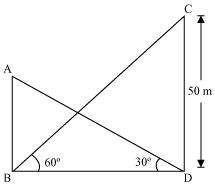Let AB be the building and CD be the tower.

In ΔCDB,

In ΔABD,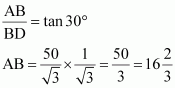Therefore, the height of the building is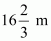.

Q10 :

Two poles of equal heights are standing opposite each other an either side of the road, which is 80 m wide. From a point between them on the road, the angles of elevation of the top of the poles are 60° and 30º, respectively. Find the height of poles and the distance of the point from the poles.

Let AB and CD be the poles and O is the point from where the elevation angles are measured. In ΔABO,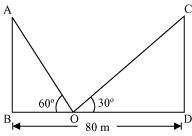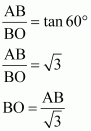In ΔCDO,

Since the poles are of equal heights, CD = AB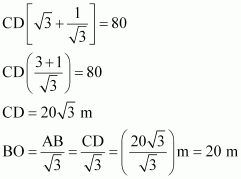DO = BD – BO = (80 – 20) m = 60 m

Therefore, the height of poles is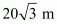and the point is 20 m and 60 m far from these poles.

Q11 :

A TV tower stands vertically on a bank of a canal. From a point on the other bank directly opposite the tower the angle of elevation of the top of the tower is 60°. From another point 20 m away from this point on the line joining this point to the foot of the tower, the angle of elevation of the top of the tower is 30°. Find the height of the tower and the width of the canal.

In ΔABC,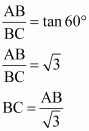In ΔABD,

Therefore, the height of the tower is m and the width of the canal is 10 m.

*Q12 :

From the top of a 7 m high building, the angle of elevation of the top of a cable tower is 60° and the angle of depression of its foot is 45°. Determine the height of the tower.

Answer :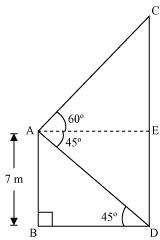Let AB be a building and CD be a cable tower. In ΔABD,

In ΔACE,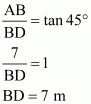AE = BD = 7 m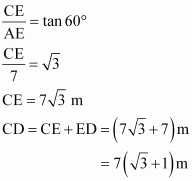Therefore, the height of the cable tower is

Q13 :

As observed from the top of a 75 m high lighthouse from the sea-level, the angles of depression of two ships are 30° and 45°. If one ship is exactly behind the other on the same side of the lighthouse, find the distance between the two ships.

Let AB be the lighthouse and the two ships be at point C and D respectively. In ΔABC,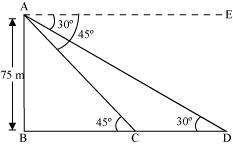In ΔABD,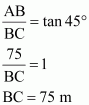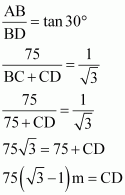Therefore, the distance between the two ships is

Q14 :

A 1.2 m tall girl spots a balloon moving with the wind in a horizontal line at a height of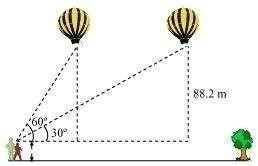88.2 m from the ground. The angle of elevation of the balloon from the eyes of the girl at

any instant is 60°. After some time, the angle of elevation reduces to 30°. Find the

distance travelled by the balloon during the interval.

Answer :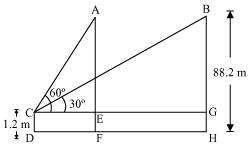Let the initial position A of balloon change to B after some time and CD be the girl. In ΔACE,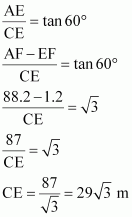In ΔBCG,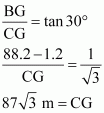Distance travelled by balloon = EG = CG – CE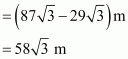Q15 :

A straight highway leads to the foot of a tower. A man standing at the top of the tower observes a car at an angle of depression of 30°, which is approaching the foot of the tower with a uniform speed. Six seconds later, the angle of depression of the car is found to be 60°. Find the time taken by the car to reach the foot of the tower from this point.

Answer :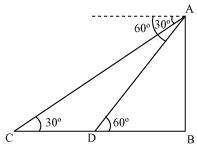Let AB be the tower.

Initial position of the car is C, which changes to D after six seconds. In ΔADB,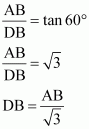In ΔABC,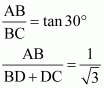Time taken by the car to travel distance DC= 6 seconds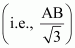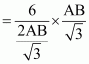Time taken by the car to travel distance DB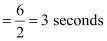Q16 :

The angles of elevation of the top of a tower from two points at a distance of 4 m and 9 m. from the base of the tower and in the same straight line with it are complementary. Prove that the height of the tower is 6 m.

Answer :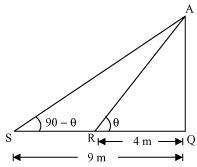Let AQ be the tower and R, S are the points 4m, 9m away from the base of the tower respectively. The angles are complementary. Therefore, if one angle is θ, the other will be 90 – θ.

In ΔAQR,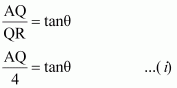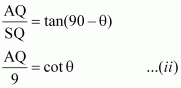In ΔAQS,

On multiplying equations (i) and (ii), we obtain

However, height cannot be negative. Therefore, the height of the tower is 6 m.

## NCERT Solutions for Class 10 Maths Chapter 9 – Some Applications of Trigonometry

For Class 10 CBSE Maths paper, out of the 80 marks, 12 marks are assigned from the chapter “ Trigonometry”. You can expect 1 question from this chapter. The paper consists of 4 parts and each carries different marks. The questions have been assigned with 1 mark, two marks, 3 marks and 4 marks. The main topics covered in this chapter include:
9.1 Introduction
In the previous chapter, you have studied about trigonometric ratios. In this chapter, you will be studying about some ways in which trigonometry is used in the life around you. Trigonometry is one of the most ancient subjects studied by scholars all over the world. As we have studied in Chapter 8, trigonometry was invented because its need arose in astronomy. In this chapter, we will see how trigonometry is used for finding the heights and distances of various objects, without actually measuring them.
9.2 Heights and Distances
The topic discusses the line of sight, angle of deviation, angle of elevation and angle of depression. All the processes are explained by solving some problems. The numerical problems are solved with the help of trigonometric ratios.
9.3 Summary
The summary describes all the points you have studied in the chapter. It will help you to understand the important concepts that need to be focused upon further from the chapter.
List of Exercises in class 10 Maths Chapter 9 :
Exercise 9.1 Solutions – 16 Questions ( 16 long answers)

In this chapter, “ Some applications of trigonometry”, class 10 students will get to know how trigonometry is helpful in finding the height and distance of different objects without measuring. In earlier days, astronomers have used trigonometry for calculating the distance from the earth to the planets and stars. Trigonometry is mostly used in navigation and geography to locate the position in relation to the latitude and longitude.

Students will learn the applications of trigonometry with real-life examples in a better way. With the help of geometrical figures, the important terms and problems are explained and the summary is given at the end of the chapter. In NCERT solutions for class 10 maths, you are provided with step by step procedure solutions of all the questions.

### Key Features of NCERT Solutions for Class 10 Maths Chapter 9 – Some Applications of Trigonometry

• The solutions are designed by subject experts.
• Access for chapter-wise questions and answers.
• Valuable guidance for the students to prepare for the board exams.
• The solutions are explained in the detailed procedure.

Conclusion

The NCERT Solutions for Class 10th Maths Chapter 9 – Some Applications of Trigonometry by Swastik Classes provide a comprehensive understanding of the various real-world applications of trigonometry. The chapter covers important topics such as heights and distances, angles of elevation and depression, and more.
The solutions provided by Swastik Classes are well-structured and easy to understand, with step-by-step explanations for all the exercises. The solutions are designed to help students develop their problem-solving skills and gain a deeper understanding of the concepts covered in the chapter.
Overall, the NCERT Solutions for Class 10th Maths Chapter 9 – Some Applications of Trigonometry by Swastik Classes are an excellent resource for students who want to excel in the subject. The solutions will not only help them score well in their exams but also prepare them for more advanced topics in mathematics.

### Where to download NCERT Solutions for Class 10 Maths Chapter 9?

NCERT Solutions for Class 10 Maths Chapter 9 can be downloaded at Swastik Classes website. It can be avail in free PDF. To download go to Swastik Classes NCERT Solutions website/select the Class 10/opt the subject as Maths/click on to the desired chapter that is Chapter 9 Some Applications of Trigonometry.

### What are the applications of learning NCERT Solutions for Class 10 Maths Chapter 9?

Applications of learning NCERT Solutions for Class 10 Maths Chapter 9 are finding the height and distance of different objects without measuring. By learning these concepts students will be able to answer all the questions based on trigonometry as well as it may help in writing class tests and board exams.

### Is NCERT Solutions for Class 10 Maths Chapter 9 enough for board exams?

Yes, you have to learn all the questions thoroughly and practice them for more time. Once you are done with solving all the questions then you can refer to other reference books and questions provided NCERT Exemplar textbooks.